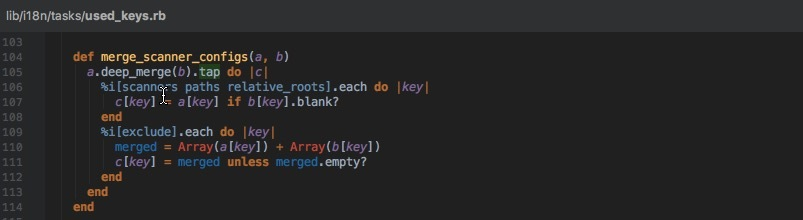#### 空指针保护

``````a ||= []
## 上面的|| 是逻辑操作符或，相当于 a || (a = [])
``````

``````class C
def data
@data ||= {}
end

def data?
@data.present?
end
end
``````

#### Tap

《ruby元编程》里面提到了tap，使用tap，可以在方法链中插入中间操作，比如：

``````temp = ['a','b','c'].push('d').shift
puts temp #=> a
x = temp.upcase.next
``````

``````['a','b','c'].push('d').shift.tap {|x| puts x}.upcase.next #=> a
````````````# source是一个Array，存放文件路径
# get_all_files 可以获取指定的单个或多个路径下所有的文件
def get_all_files(source)
files = []
source.map do |path|
path = path[-1] == '/' ? path : path + '/'
group = Dir.glob("#{path}**/**")
files << group.reject { |x| File.directory?(x) }
end
end
``````

``````def get_all_files(source)
[].tap do |files|
source.map do |path|
path = path[-1] == '/' ? path : path + '/'
group = Dir.glob("#{path}**/**")
files << group.reject { |x| File.directory?(x) }
end
end
end
``````

#### Symbol#to_proc

``````a = [1,2,3,4]
a.reduce(&:+) #= > 10
a.inject(0,&:+) #= > 10
``````

「reduce 和inject 是alias的关系, 两者可以互用。我依然记得第一次看到数组求和`[1,2,3,4].reduce(&:+)` 这个例子时，一脸懵逼……」

``````a = [1,2,3,4]
a.reduce{|sum, x| sum + x } #= > 10
a.inject(0){|sum, x| sum + x } #= > 10
``````

``````b = ['hi','ruby','hello']
b.map(&:capitalize) #= > ["Hi", "Ruby", "Hello"]
b.map(&:nil?) #= > [false, false, false]
b.map(&:concat('world')) #= > raise: syntax error
``````

b中的每一个元素都是String对象，可以成功调用无参的实例方法capitalize，nil?等，但是当调用带有参数的concat方法时就会报错，为什么？

``````class Symbol
def to_proc
Proc.new {|x| x.send(self)} # 动态派发，调用self这个method的
end
end
``````

#### 参考

《ruby元编程第二版》

Ruby: Tap that method

Understanding Ruby’s idiom: array.map(&:method)